SEARCH HOMEMath Central Quandaries & QueriesQuestion from DIANNE, a student: 5(16-9) divided by 7x2+14 top part of equation _____________________________________ 12-3x2 bottom partHi Dianne,

Let me try a similar problem,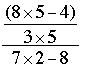I am going to evaluate the three parts separately

8 × 5 - 4: We always multiply before adding so this is 40 - 4 = 36.
3 × 5: This is 15.
7 × 2 - 8: Again, multiply first and get 14 - 8 = 6.

Thus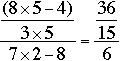What I see now is that 3 divides 36 (36 = 3 × 12) and 3 divides 15 (15 = 3 × 5) and thus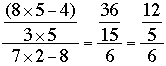But this is one-sixth of 12 divided by 5 and hence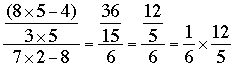Finally both 6 and 12 are divisible by 6 and thus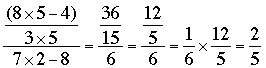Now try your problem and write back if you need more help.

PennyMath Central is supported by the University of Regina and The Pacific Institute for the Mathematical Sciences.